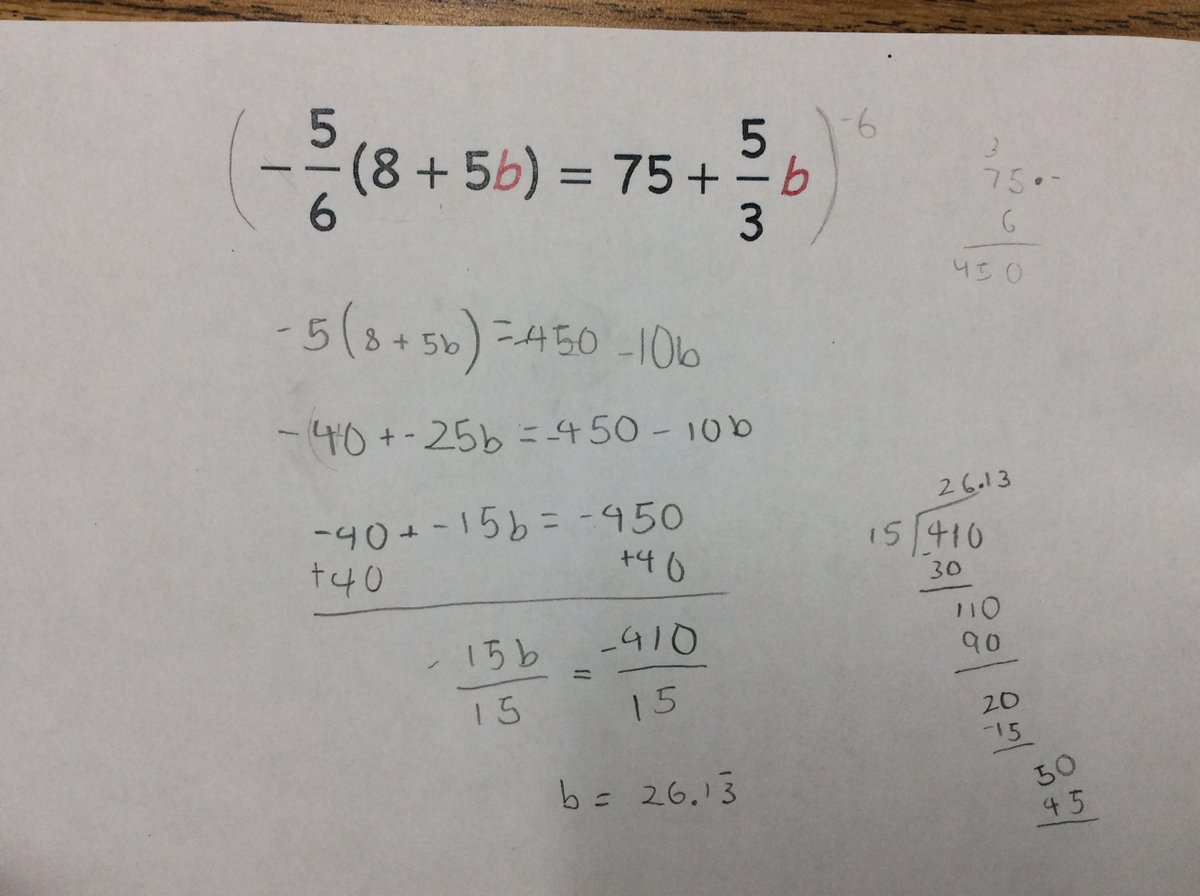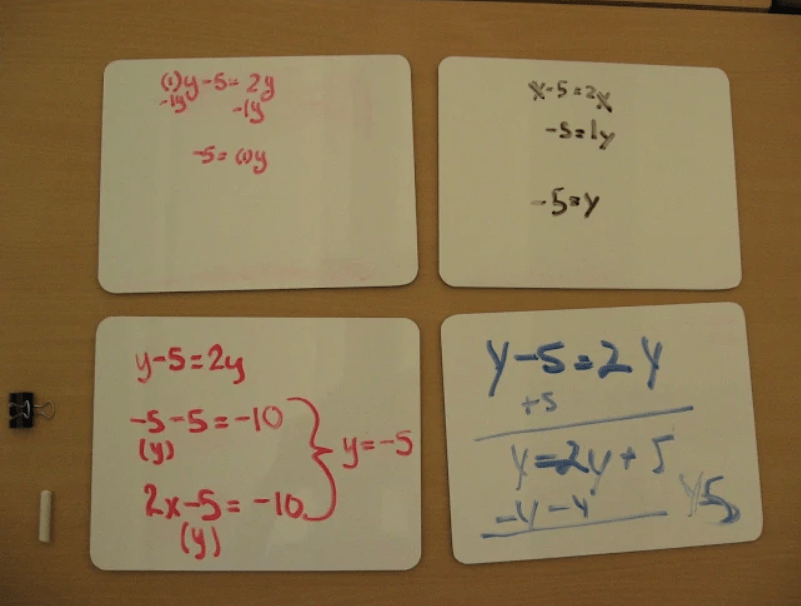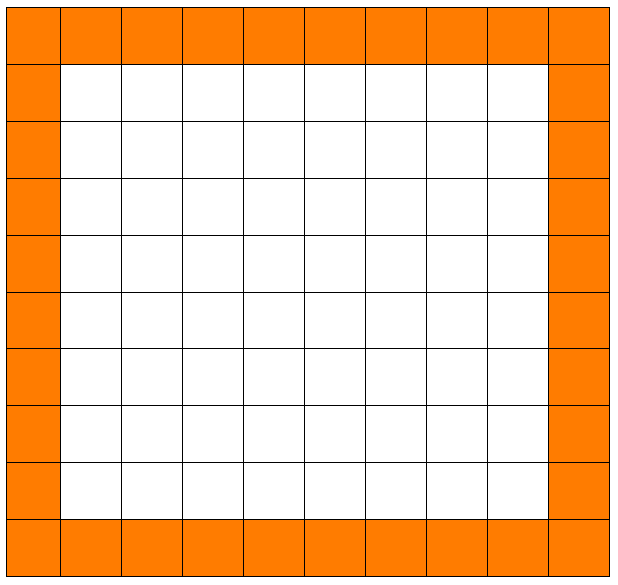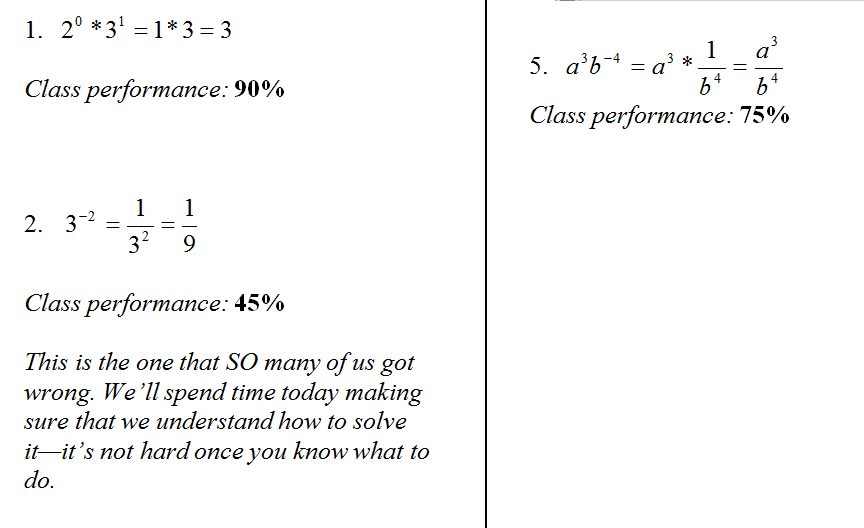Categories

## Dear Diary: What types of equations are hard to solve?

I’ve been begging people lately to send me their solving linear equations mistakes. As long as people indulge me, this will be a series of posts devoted to thinking aloud about what sorts of linear equations are hard to solve, and what it is that makes them hard, and what exactly it means to know how to handle every equation that’s out there. The goal I have in mind is a way to be able to teach a (relatively) new teacher what sorts of equations they can expect their students to struggle with and (ideally) how to systematically teach this stuff so that kids see all the cases. (Besides for the obvious, e.g. an equation with lots of stuff in its that requires a ton of steps).

I don’t have settled thoughts about this, but I do need a place to work this stuff out. These posts are unfinished and sketchy. It’s basically my math mistakes diary. You should only read this if that sounds like fun to read…

…and here we go!

***

I think there are a lot of things that make this hard. I don’t know about Michelle’s students, but fraction multiplication is touch-and-go with my students, so there’s that factor.

One of the most important little “micro-skills” relating to equations that I teach my students is about scaling equations up so as to eliminate fractions. I think that would be useful here.

There’s an interesting choice about whether to do that scaling before or after distributing. That’s another interesting little choice that a student would have to make. I could imagine designing a little activity centered on a bit of strategy: when does it make sense to scale up? I think the message to kids could be that if you scale up before distributing, you might get to avoid some fraction arithmetic.

Maybe the activity could look like this. You start with that equation and then you present two options.

“Amanda took that equation and did this: 14m – 7 – 3m/5 = 24/5 – 18m/3”

“Billy’s first move looked like this: 35(2m – 1) – 3m = 6(4 – 3m)” [include annotations to make it clear what Billy did!]

And then you offer some prompts to draw attention to the choices you get to make?

Micro-skills here: knowing whether to distribute first, or whether to wait; scaling up an equation to avoid fractions

***

Awesome!Just be explicit about the mistake…we scale both sides by -6, but the left side ends up only getting scaled by 6. The fractions seem sort of incidental to this one. Maybe this is related to the thing where multiplying by a negative feels like it should make the stuff negative, rather than giving everything its opposite sign?

To what extent is this an equations mistake vs a negatives mistake?Lots going on here. The idea that we’re scaling both sides vs. just the fractions is tricky it seems. Clearly fractions make an equation tricky to solve as it presents a number of conceptual issues. To what extent is it helpful for a teacher to understand anything about how kids handle equations with fractions besides “with difficulty”? It seems like all the interesting choices to make when handling equations with fractions are about finding ways to transform that equation so that we can avoid those fractions.There’s an interesting choice to make about whether you try to multiply both sides of an equation by 2 or by -2. What makes that interesting is again that multiplying by a negative introduces conceptual issues that aren’t introduced by x2. I feel like I’m getting close to saying that, as a matter of coaching, it makes sense to encourage kids to scale equations by positive numbers whenever possible. (Which, I think, is always.)

Micro-skills involved: What do you choose to scale each side of the equation by? When do you choose to scale each side of the equation? (Do you distribute first, or scale first?)

***

This was one of mine that I wrote about elsewhere:

Yesterday I gave students a no-grades quiz in algebra. A student who, I had been told at the start of the year, frequently struggles in math, has been having a lot of success lately. She knew exactly how to handle both of the systems of equations that were on this short quiz, but she got stumped at one of the resulting equations:$-1.7x = 4.3x + 3.6$

I didn’t know what to say when she got stuck, exactly, but I was fairly confident that this was an example of a micro-skill that she was missing.

She and I agreed that she’d like me to write a little example on the side of her page, so I wrote this:$-2x = 5x + 7$

[I drew some arrows going down from each side labeled “+2x.”]$0 = 7x + 7$

My student read the example and then exclaimed (in a way I can only describe as “joyous”), Oh wait, you can make 0 there?!

Micro-skills: Knowing that you can make 0.

There seem to be a bunch of cases where you introduce and manage zeroes in solving equations. Another one of mine that I have rattling around in the archives:Check out the bottom right. This pair of students got frozen at y = 2y + 5 because they subtracted y from each side and then weren’t sure if they were allowed to end up with 0. (Two years ago I talked to these kids about this, so I’m not guessing from their work.) This seems identical to the situation that this year’s student.

The moral of the story to me is that making zeroes is something that students need “permission” to do. Not like permission from me, the mathematical authority. I just mean that it seems to be something that beginners need help realizing is a kosher move.

As long as this picture is here, another little decision that kids need to make (see top-right) is whether to add or subtract something when trying to use a balancing move.

Microskill: “making a zero” to decide whether to add/subtract something from each side of equation

Categories

## The Border Problem and Variables

In this 7th grade class, they’re studying interesting ways of counting up the boxes in the border of a square grid.They originally start with a 10 by 10 grid, but soon after they expand to a  6 by 6 gride and a 15 by 15 grid and use their techniques to count the squares in the new border. Then, in class, they introduce the notion of variable and set about shortening one of their verbal explanations using variables.

On the board are two expressions: 6 + 6 + 4 + 4 and 15 + 15 + 13 + 13. The teacher tasks the students with writing an algebraic expression that represents the number of squares on the border. The following is a recorded interaction among students during their group work:

Sharmeen: s + s + (s-2) + (s-2). Though that’s kind of complicated. Is there any other way to put it?

Antony: What is it?

Sharmeen: Uh, mine? It was s + s + (s-2) + (s-2)

Kim: No we had to, like, um, how about we write a variable for…make a variable for thirteen.

Sharmeen: Yeah, oops. Oh, m equals… OK, so it’s s + s + m + m.

What make you of this?

[All of this is lifted from “Connecting Mathematical Ideas“.]

Categories

## Negative powers, with numbers and exponentsI just dug this up. It’s what I handed back students after a “pre-quiz” (i.e. a quiz at the end of the unit, but before their quiz). I had forgotten that during that first year I handed back these things with class performance percentages on them.

Anyway, the way those percentages break down is interesting to me. Is it surprising that kids had so much trouble with negative exponents in numerical context, but had such less trouble with variables?

Categories

## Solving Equations Whoopsies

Comment on anything that you like, but here are some prompts:

• Do you like my questioning? (Because I didn’t. Notice that moment when I pause and try to unask my question?)
• Describe, as best you can, the way that this kid thinks about the Distributive Property.
• What would you say next, if you were me?
• Does this video have implications about the way that you’d teach this topic? (And, come on, don’t give me that “they need lots of practice stuff.” Of course they do. But what else?)
• Do you prefer having videos over images on this site? (Because I have about a half hour more footage from my work with this kid…)

Looking forward to a great bunch of comments here. Don’t let me down?

Update:

Here’s how I responded:

Categories

## Solving Simple EquationsWhat’s the quickest way that you could help this student?

Thank you to Anne Bailey for the submission!